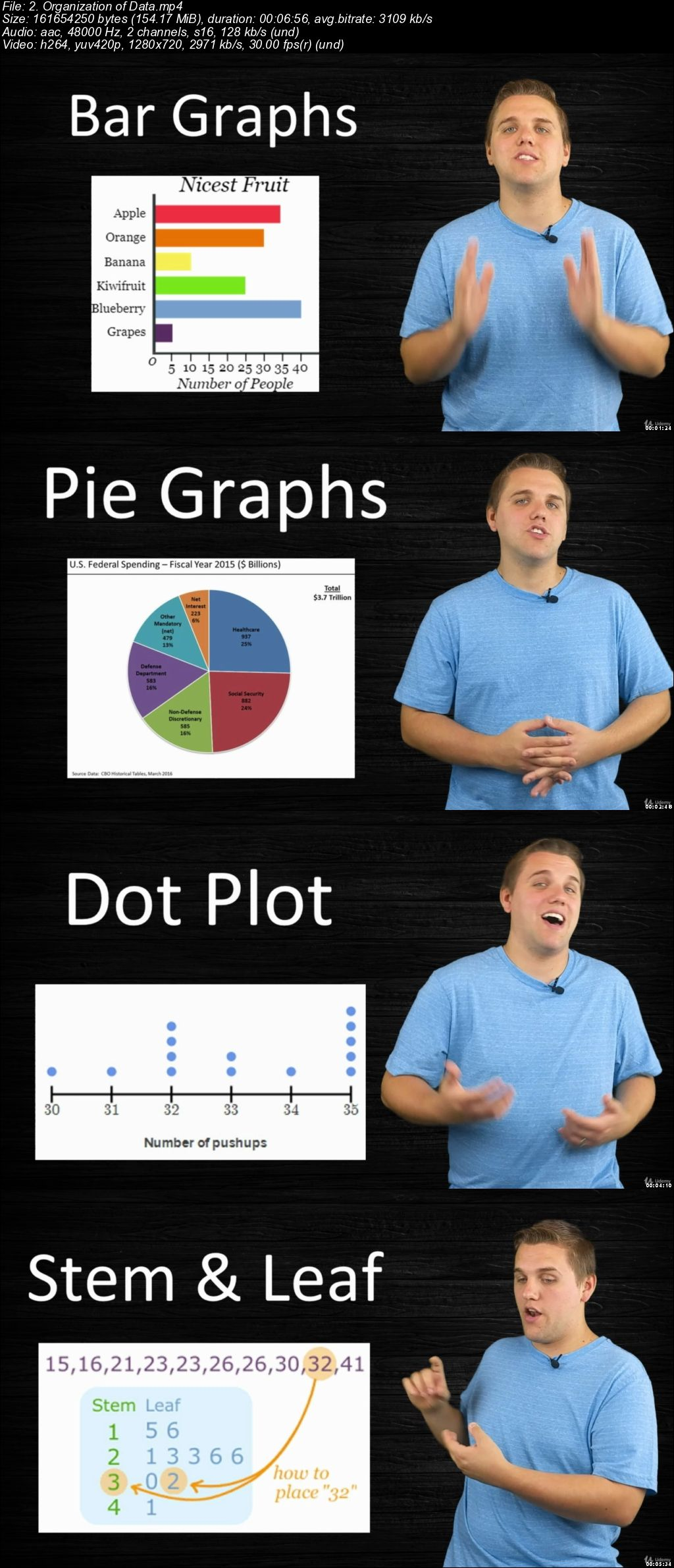# Statistics & Probability (Complete) For CollegeAP StudentsStatistics & Probability (Complete) For College/AP Students
.MP4 | Video: 1280×720, 30 fps(r) | Audio: AAC, 48000 Hz, 2ch | 16.2 GB
Duration: 13.5 hours | Genre: eLearning | Language: English

Includes 100+ Meaningful Lectures, Homework Assignments, Quizzes, Comprehensive Exams & An Online Statistics Textbook!

What you’ll learn

College Statistics teaches skills in understanding whether data is meaningful, including optimization, inference, testing, and other methods for analyzing patterns in data in ways that are useful across all fields of study.

Requirements

You should have some understanding of basic arithmetic.
Other than that, this course starts from the building blocks of statistics and probability, and works from there!

Description

This course has been updated in 2019. Check out some of our lectures below!

Included in the purchase of this course are:

100+ Comprehensive & Meaningful Lectures

Dozens Of Homework Assignments (Autograded) Via MyOpenMath

Several Quizzes (Autograded) To Assess Understanding Of Each Section

Comprehensive Midterm Exams And Final Exams (Autograded) To Assess Understand Of The Entire Curriculum

A Complete Open-Source Online Statistics Textbook

You are probably asking yourself the question, "When and where will I use statistics?" If you read any newspaper, watch television, or use the Internet, you will see statistical information. There are statistics about crime, sports, education, politics, and real estate. Typically, when you read a newspaper article or watch a television news program, you are given sample information. With this information, you may make a decision about the correctness of a statement, claim, or "fact." Statistical methods can help you make the "best educated guess."

Since you will undoubtedly be given statistical information at some point in your life, you need to know some techniques for analyzing the information thoughtfully. Think about buying a house or managing a budget. Think about your chosen profession. The fields of economics, business, psychology, education, biology, law, computer science, police science, and early childhood development require at least one course in statistics.

In this course, you will learn:

Recognize and differentiate between key terms.

Apply various types of sampling methods to data collection.

Create and interpret frequency tables.

Display data graphically and interpret graphs: stemplots, histograms, and box plots.

Recognize, describe, and calculate the measures of location of data: quartiles and percentiles.

Recognize, describe, and calculate the measures of the center of data: mean, median, and mode.

Recognize, describe, and calculate the measures of the spread of data: mean average deviation, standard deviation, and range.

Understand and use the terminology of probability.

Determine whether two events are mutually exclusive and whether two events are independent.

Calculate and interpret expected values.

Recognize and understand continuous probability density functions in general.

Recognize the normal probability distribution and apply it appropriately.

Recognize the standard normal probability distribution and apply it appropriately.

Compare normal probabilities by converting to the standard normal distribution.

Recognize central limit theorem problems.

Classify continuous word problems by their distributions.

Apply and interpret the central limit theorem for means.

Apply and interpret the central limit theorem for sums.

Calculate and interpret confidence intervals for estimating a population mean and a population proportion.

Interpret the Student’s t probability distribution as the sample size changes.

Discriminate between problems applying the normal and the Student’s t distributions.

Calculate the sample size required to estimate a population mean and a population proportion given a desired confidence level and margin of error.

Describe hypothesis testing in general and in practice

Conduct and interpret hypothesis tests for a single population mean, population standard deviation known.

Conduct and interpret hypothesis tests for a single population mean, population standard deviation unknown.

Conduct and interpret hypothesis tests for a single population proportion.

Some content was used from Creative Commons, and attribution is provided within the curriculum of this course.

Who this course is for:

College Statistics follows the scope and sequence requirements of a one-semester statistics course and is geared toward students in AP Statistics or any college-level statistics course.